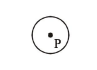# A perfectly dimagnetic sphere has a small spherical

Question:

A perfectly dimagnetic sphere has a small spherical cavity at its centre, which is filled with a paramagnetic substance. The whole system is placed in a uniform magnetic field $\overrightarrow{\mathrm{B}}$. Then the field inside the paramagnetic substance is:1. Zero

2. $\overrightarrow{\mathrm{B}}$

3. much large than $|\overrightarrow{\mathrm{B}}|$ but opposite to $\overrightarrow{\mathrm{B}}$

4. much large than $|\overrightarrow{\mathrm{B}}|$ and parallel to $\overrightarrow{\mathrm{B}}$

Correct Option: 1

Solution:

A perfect diamagnetic substance will completely expel the magnetic field. Therefore, there will be no magnetic field inside the cavity of sphere. Hence the paramagnetic substance kept inside the cavity will experience no force.